One can straightforwardly divide the integration domain into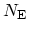equidistant intervals. A disadvantage of this method is that the numerical error can not be pre-defined. This problem is more pronounced when the integrand is not smooth. To evaluate (4.60) numerically a trapezoidal rule and an equidistant grid spacing are used. The dependence of the accuracy on the following two parameters is studied, namely the grid spacing,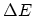, and the relative distance between the peak and the nearest grid point,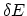. These parameters are normalized as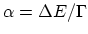and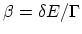. The relative error in calculating the carrier concentration,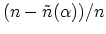, as a function of grid spacing is shown in Fig. 4.10. Here,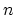is the analytically exact value of the carrier concentration (4.60) and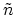refers to the numerically calculated carrier concentration as a function of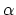and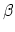.
The variation of the calculated carrier concentration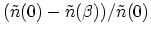with respect to the shift of energy points is shown in Fig. 4.11. The reference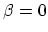implies that one of the grid points aligns with the peak of the resonance. The oscillatory behavior depends on the grid spacing. A shift equal to the grid spacing gives the same result. As a measure of the sensitivity of the calculated carrier concentration with respect to grid positions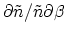is shown in Fig. 4.12. To reduce this sensitivity, a very fine grid spacing has to be adopted. This quantity is characteristic of the numerical error, and needs to be controlled to avoid convergence problem in the self-consistent iteration loop (see Section 4.9.2).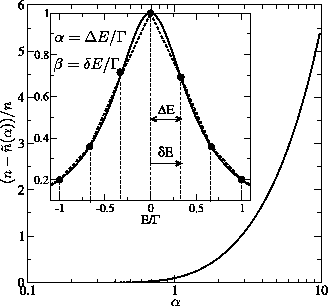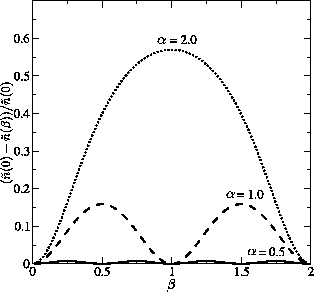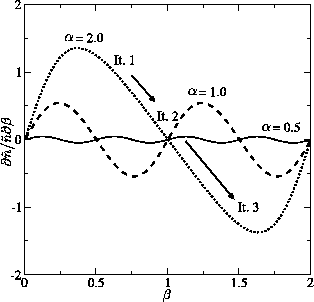In summary, the accuracy of the non-adaptive method strongly depends on the grid spacing and the position of grid points. If the grid spacing is sufficiently fine,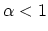, the numerical error is small, but it increases considerably for coarser grid spacing,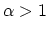. For accurate results a grid spacing smaller than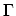has to be employed. For example, to resolve a resonance of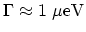width in an energy range of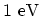more than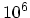energy grid points are required, which would severely increase the computational cost. For even narrower resonances, (eg.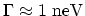), an equidistant grid is no longer feasible. To avoid these problems an adaptive method needs to be employed. M. Pourfath: Numerical Study of Quantum Transport in Carbon Nanotube-Based Transistors